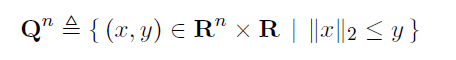# How to express 'if x<0, x==x else x==0' in the cone? such as in lorentz

How to express ‘if x<0, x==x else x==0’ in the cone? such as in lorentz

The definition of a Lorenz cone (say in 3d) is

so it automatically imposes that x,y\geq 0.

If you were interested in something else then I think you have to provide more context for what you want to achieve.

I check the definition of Lorenz cone in CVX, like this:Now, I have a cone like this:
{ [x(1);x(2);x(3);x(70);x(71);x(72);],F} lorentz(6)

I want to minimize the norm of the frist six elements. Now I pay more attention to x(1). I just want to minimize it when x(1) <0. That means, whatever how larger it is when x(1)>0, I do not care. I do not know how to express it? Write a new function?
Thanks

If I understand correctly then you should use max(-x,0) instead of x for each variable x which you want to treat like that.